# How do array formulas work in Google Sheets?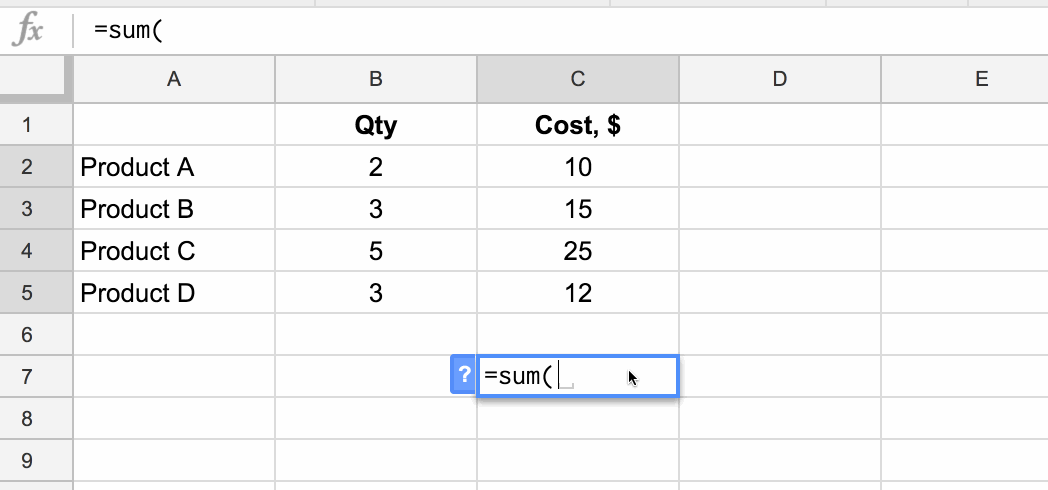In this post I’m going to run through the basics of using array formulas, and you’ll see they’re really not that scary. Hip, Hip Array!

## What are array formulas in Google Sheets?

First of all, what are they?

To the uninitiated, they’re mysterious. Elusive. Difficult to understand. Yes, yes, and yes, but they are incredibly useful in the right situations.

Per the official definition, array formulas enable the display of values returned into multiple rows and/or columns and the use of non-array functions with arrays.

In a nutshell: whereas a normal formula outputs a single value, array formulas output a range of cells!

The easiest way to understand this is through an example.

Imagine we have this dataset, showing the quantity and item cost for four products: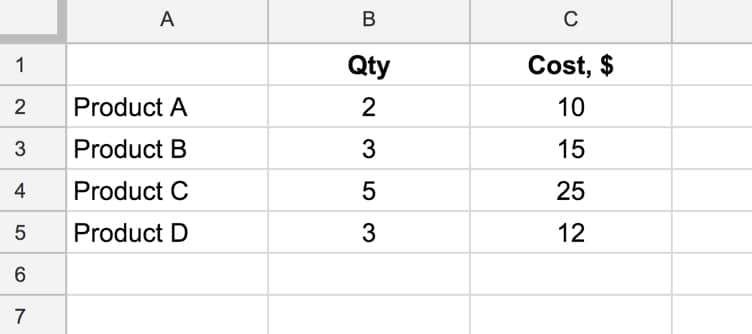and we want to calculate the total cost of all four products.

We could easily do this by adding a formula in column D that multiplies B and C, and then add a sum at the bottom of column D.

However, array formulas let us skip that step and get straight to the answer with a single formula.

### What’s the formula?

`=ArrayFormula(SUM(B2:B5 * C2:C5))`

### How does this formula work?

Ordinarily, when we use the multiplication (*) operator in a Sheet, we give it two numbers or two cells to multiply together.

However, in this case we’re giving it two ranges, or two arrays, of data:

`= B2:B5 * C2:C5`

However, when we hit Enter this gives us a #VALUE! error as shown here: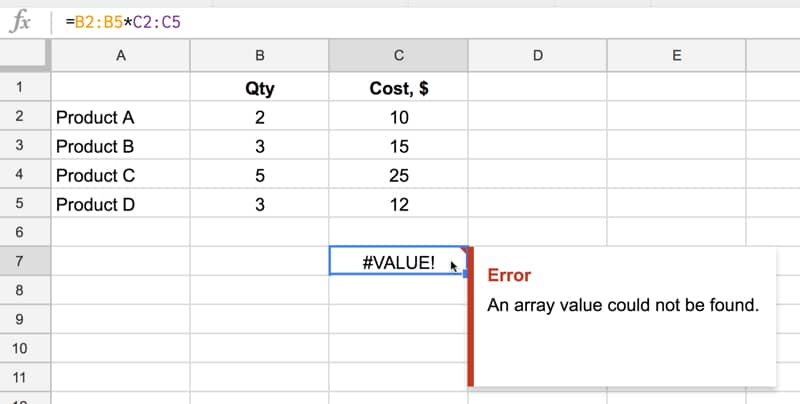We need to tell Google Sheets we want this to be an Array Formula. We do this in two ways.

Either type in the word `ArrayFormula` and add an opening/closing brackets to wrap your formula, or, more easily, just hit Ctrl + Shift + Enter (Cmd + Shift + Enter on a Mac) and Google Sheets will add the ArrayFormula wrapper for us.

`=ArrayFormula(B2:B5 * C2:C5)`

Now it works, and Google Sheets will output an array with each cell corresponding to a row in the original arrays, as shown in the following image:

Effectively what’s happening is that for each row, Google does the calculation and includes that result in our output array (here showing the equivalent formulas):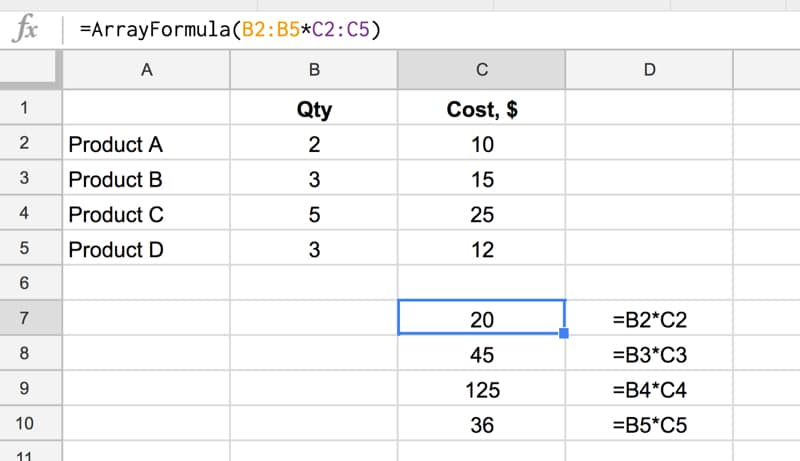and another view, showing how the calculation is performed (just for the first and last row):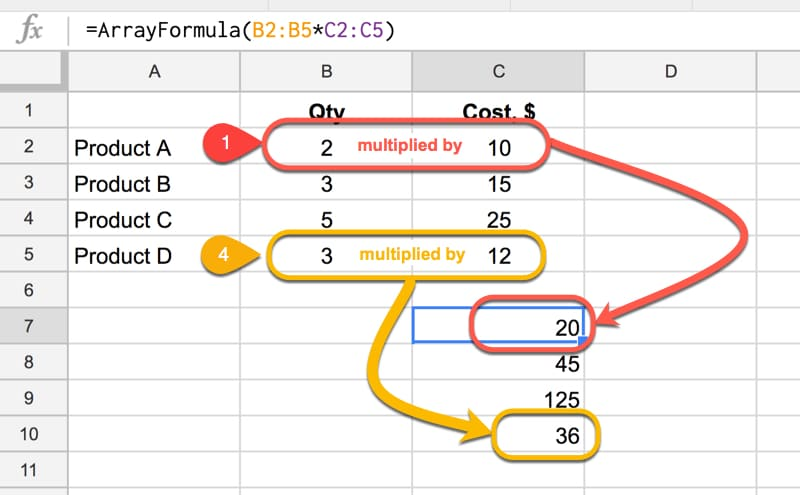Note: array formulas only work if the size of the two arrays match, in this case each one has 4 numbers, so each row multiplication can happen.

Finally, we simply include the SUM function to add the four numbers:

`=ArrayFormula(SUM(B2:B5 * C2:C5))`

as follows:Quick Aside:

This calculation could also be done with the SUMPRODUCT formula, which takes array values as inputs, multiplies them and adds them together:

`=SUMPRODUCT(B2:B5 , C2:C5)`

### Another Array Formula Example

In this example, we enter a single formula to multiple an array of row headings against an array of column headings. Again, this only works because the two arrays are the same size: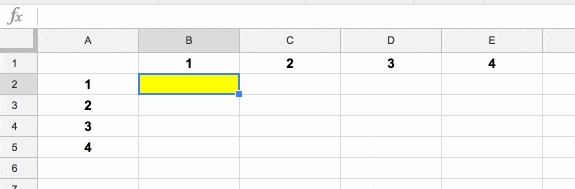## 73 thoughts on “How do array formulas work in Google Sheets?”

1.Mike Booth says:

Hi Can you use arrayformulas wth text and numbers? I have a spreadsheet that then goes on to create a look up table and the I have copied down the formula =C3&A3 to create, for example VAN1 however I inserts rows into the sheet and I am trying to find a solution to automatically copy the formula. I think it should be an array formula however having spent 3 hours searching for a solution i’m, struggling – here is the sheet https://docs.google.com/spreadsheets/d/1HJFGX_4vhcGMefmO0fhDFZHnFcH6APdr_3-M7YNHgz4/edit?usp=sharing

1.Ben says:

Hey Mike,

Looks like you’ve got the array formula working now? You should be able to do it with something like this:

`=ArrayFormula(C:C&B:B)`

Also, you should change the permissions on your sheet to View-only or turn sharing off again, in case anyone else clicks that link.

Cheers,
Ben

2.Jay says:

OR & AND functions do not work inside the otherwise awesome ARRAYFORMULA. Is there an easy way to substitute OR & AND inside the ARRAYFORMULA?

1.Pedro says:

What I did to overcome this with the AND() formula was to substitute it by a multiplication (*), so:

=AND(TRUE, TRUE)

is equivalent to writing:

=TRUE * TRUE

1.Ben says:

Great tip! Thanks for sharing Pedro 🙂

2.Monica says:

Pedro, you are my hero! I spent hours last night trying to make AND formula work within ARRAYFORMULA and you solved it for me. Thanks!
Ben: you are my hero too, I’ve learned a lot from you. 🙂

2.Mark says:

I have a google spreadsheet where I have the first row as (for example):

`=googlefinance("AAPL","price",today()-60,today())`

The historical data actually only returns 60 days of data up to yesterday, and the number of rows varies with weekends/holidays.

So I manually have to place the following at the next empty row following whatever that above formula returns to return the current price for today:

`=googlefinance("aapl")`

Since the number of rows returned for the first formula varies, I have to delete the last one, let the first formula populate rows, and then place the last formula on the next empty row.

Can I use arrayformula (or something else) to basically append the last formula to the result set of the first formula?

1.Ben says:

Hey Mark,

This formula should do the trick for you:

`={googlefinance("AAPL","price",today()-60,today());{today(),googlefinance("AAPL")}}`

which uses an array formula to append the current result to the historic one.

Hope that helps.

Cheers,
Ben

3.miriam fink says:

What arrayformula do I use if I want to use the value in A and multiply by diff amounts. For example : A multiplied by 4 for row B, A multiplied by .5 for row C, etc.

4.Reggie says:

What would be the syntax if I wanted to use an array formula for the summation of 3 columns together?

1.Ben says:

You can use a standard SUM formula to sum 3 columns together, e.g. this will add all of A, B and C together:

`=sum(A:A,B:B,C:C)`

1.Kees Huizing says:

I have this with 10 columns. Of course I could write
=sum(A:A,B:B, …., J:J)
but that is a bit tedious. In a non-arrayformula I would write
=sum(A1:J1)
and I need more of these on other column ranges. Is there a way to use array formulas without having to mention each column individually?

1.Stu says:

What does =sum(A:A,J:J) produce as an array formula?

5.Kevin Flavin says:

Hi Ben,

Love your work, really saves a ton of time. Thank you.

Here’s the prob: I have dates in the first column, and the second column is an index value, say {A,B,C}, so each date has three index corresponding to them, and the remaining 10-15 columns contain information, e.g. shirt size, color, cost, price, source, etc.

How can I find the color (column 4) by matching the date and the index value, say “B”?

This is a typical database-style query, but I don’t want to make a database for one spreadsheet.

Thanks!!

1.Ben says:

Hi Kevin,

Not exactly sure what you’re after, but it sounds like you might need a lookup based on multiple conditions. Have a look at this post from Chandoo’s site: https://chandoo.org/wp/2010/11/02/multi-condition-lookup/

Hope that helps!

Ben

6.Taylor Tholen says:

I’m not sure if this is the proper use of Arrayformula but I have a sheet that I clear with a script (which wipes out all the formulas) and need to add back the formulas with another script. Am I headed in the right direction, or is this even enough info?

1.Ben says:

Hi Taylor,

Sounds like you can use Apps Script to create your new formulas, so it doesn’t sound like you’d need the ArrayFormula.

Ben

2.Manish Prasad says:

Hi Taylor,

Can you help me with the script which wipes out all the formulas and then after a defined time it adds back the formulas again.

7.Patrick Blood says:

Good evening, Ben.

I have looked all over and I can’t seem to find an easy solution to this issue of my linked Forms-to-Sheets Query function adding a pesky extra row. Because of this, my form (response) data never gets moved over (via the Query function) and calculated. Help!

So, I have the ‘Form Responses’ tabbed sheet and I have created a separate tab within that sheet that I call ‘Calculations’. In Calculations I am placing =ARRAYFORMULA(Query(‘Form Responses’!A2:AE2)) in cell A2. When the form is filled out and submitted, responses properly fill my A2:AE2 cells in ‘Form Responses’ but, when I jump over to ‘Calculations’, there is no data within the row. What is frustrating is that the A2:AE2 in my argument now says A3:AE3. The more responses I receive the higher the number; A3:AE3 becomes A4:AE4 and so forth. I have also just tried the Query command alone without the ARRAYFORMULA and I still run into this problem of Sheets wanting to add this invisible row. Is there a fix? If so, could you help me out?

1.Ben says:

Hi Patrick,

You shouldn’t need the ArrayFormula in this example. The query function deals in arrays already.

Try changing your query function to this:

`=QUERY ( A1:AE , "select *", 1)`

You need to have a select clause in there and also leave off the final row reference, like this A1:AE.

Hope that helps!

Ben

8.Carmella says:

I hope you can help. I am trying to use the copydown add on for a =vlookup command. I am using a separate sheet to lookup an email address when a form is completed with a specific school.
Is this possible to do with an Array Formula?

1.Ben says:

Yes, you can nest your vlookup inside an array formula, for example:

`=ArrayFormula(if(A1:A<>"",vlookup(A1:A,Sheet2!\$A\$1:\$B\$5,2,false),""))`
9.Roberto Tognelli says:

Hi, Ben! Is it possible to use ArrayFormula to fill a column with, in each cell, the sum from that cell to the bottom end of the column?
Many thanks!

10.Jorge Gonzalez says:

Hello I have a =getStatusCode(D3) which I have to manually copy or drag down

is there a way I can add this code to the column automatically and that it updates ex. to D4, D5,D6 etc?

Here’s the code for the script editor:

Utilities.sleep(Math.random() * 1000);
function getStatusCode(url)

{
var url = encodeURI(url)
var options = {

‘muteHttpExceptions’: true,
‘followRedirects’: false
};

var response = UrlFetchApp.fetch(url, options);
return response.getResponseCode();
}

1.John says:

Maybe my later Comment on UDF’s on
September 7, 2018 at 12:53 pm
entitled
The issue described here may be of interest to those who use ARRAYFORMULAS and need to combine with UDF’s.
…..
may be of use.
John

11.Stone says:

I’m trying to combine the fill down idea with the google finance function like this:

column K is a dynamic ticker list that’s populated by a query, but this formula only finds the ticker in k2. How do I get it to find k3, k4, etc.?

12.Angel says:

Is it right that Arrayformula and the IF function don’t work together in google sheets?

13.sifar says:

I have a spreadsheet which displays the Current Month if the Current Month matches the Month of the date in Column A. If the dates in Column A do not match with Current Month, then i would like to display the Previous Month, but not 2 or more months. At a time, only 1 month should be present in the Current Month column (either Current Month OR Previous Month), otherwise it should be blank.

for some reason, this ArrayFormula evaluates and shows both the Current as well as Previous months.

=ARRAYFORMULA(IF(A2:A = “”, “”, IF(TEXT(DATE(YEAR(B2:B),MONTH(B2:B),1),”mmm-yyyy”) = TEXT(DATE(YEAR(TODAY()),MONTH(TODAY()),1),”mmm-yyyy”), UPPER(TEXT(DATE(YEAR(TODAY()),MONTH(TODAY()),1),”mmm”)), IF(((TEXT(DATE(YEAR(TODAY()),MONTH(TODAY())-1,1),”mmm-yyyy”) = TEXT(DATE(YEAR(B2:B),MONTH(B2:B),1),”mmm-yyyy”))), UPPER(TEXT(DATE(YEAR(TODAY()), MONTH(TODAY())-1, 1),”mmm”)), “”))))

How can this formula be modified to list only either Current or Previous month?

14.Tim says:

Seems like this would be simple for what I am trying to do with the arrayformula function. I have an event form that the event coordinator takes down event information from customers. I need the column that shows what day of the week to parse the XX/XX/XX and the result would be “Saturday”. I have that formula working no problem but when an new entry is submitted it drops the row down. Thinking arrayformula would work but I cant get it to be consistent in the column.

15.Cathrynn says:

Hi there, I am trying to create an Order Form. Some items are different prices and different quantities. Example

Item 1
Blue (\$8) – 1 unit
Pink (\$12) – 2 units

Will this formula work and can I display the amount in the form before submission?

Thank you!!

16.Alex says:

Hi, Ben!

How could I run this with ArrayFormula. I’ve read I have to replace “OR” with “+” but I can’t really wrap my head over it. Thank you!

=IF((or(B51=”Austria”, B51=”Finland”, B51=”France”, B51=”Germany”)),”EUR”,if(B51=”Denmark”, “DKK”, if(B51=”Norway”, “NOK”, if(B51=”Sweden”, “SEK”, if(B51=”United Arab Emirates”, “AED”, if(B51=”United Kingdom”, “GBP”, if(B51=”United States”, “USD”,”NA”)))))))

1.John says:

In reply to Alex. Look at my comment in the original article on using OR or + in a FILTER function which can usually be avoided.
There are also much better ways of handling multiple OR’s.

See here for a way of avoiding + by using MATCH in a situation where matching is actually the task!

17.John says:

The issue described here may be of interest to those who use ARRAYFORMULAS and need to combine with UDF’s.
An issue with user defined functions (UDF’s) and indeed some inbuilt sheet functions such as CELL when used with ARRAYFORMULA is that they don’t deal with array ranges properly or as you would like is probably more accurate to say.

For example “=ARRAYFORMULA(CELL(“address”,A1:C1))) only provides a 1-element array \$A\$1 rather than the array [\$A\$1,\$A\$2,\$A\$3] which is presumably what might be desired. The reason being that such functions are not designed to work with arrays.

I needed a function which would automatically provide the column labels A,B etc. as an array for a range rather than just column numbers. For example I wanted to use something like “=TRANSPOSE(ARRAYFORMULA(colLabel(COLUMN(someRange))))” where colLabel is a UDF which returns the column label given the column number. This unfortunately doesn’t work as also CELL doesn’t unless you make provision for dealing with an array of values rather than a single value passed to your UDF.

I couldn’t find anything which deals with this issue simply so I came up with the following which should also indicate how to proceed whenever you need to provide a UDF which can be used with ARRAYFORMULA.

function colLabel(rngValues){
// Function which processes the individual cell values passed to the UDF
function label(a){
var temp, l = ”;
while (a > 0)
{
temp = (a – 1) % 26;
l = String.fromCharCode(temp + 65) + l;
a = (a – temp – 1) / 26;
}
return l;
}
// Basic handler for the passed 2D range values
// Process the array values with a function like “label” to suit your requirements
// rngValues is a Javascript 2D array
for(var j=0;j<rngValues.length;j++){
// r is row j of the array rngValues which contain the row values in array r=rngValues[j]
var r=rngValues[j]
for(i=0;i<r.length;i++){
// Press the row values and pass the new values back
r[i]=label(r[i]) }
}
// Return the processed array
return rngValues
}
HTH.

18.Mary says:

Hi Ben,

Your site really help me, thank you.

I’ m working on a GoogleSheet with four columns with dates (A,B,C,D)

I have the column A to choose a date, and this formula on the columns B,C,D to change automatically two weeks :

=ARRAYFORMULA(IF(A1:A””; TEXT(TO_DATE(DATEVALUE(A1:A) + 14);”yyyy/mm/dd”);””))

But now I need to change the date on column B manually and want to C and D change automatically. But logically I have an error formula. There’s a possibility to do this without GoogleScript?

Thank you again
Mary

19.Allan Bech says:

Hi Ben.

Can you help figure this out; (TÆL.HVIS = COUNTIF and ; = , in danish)

=ARRAYFORMULA((TÆL.HVIS(\$A4:\$O4;”under middel”)*(0)+(TÆL.HVIS(\$A4:\$O4;”middel”)*(1))+(TÆL.HVIS(\$A4:\$O4;”over middel”)*(2)))/(TÆL.HVIS(\$A4:\$O4; “*middel*”)))

I can only make this happen in its own cell and not the column. What am I missing?

Thank you…
Allan

20.Peter says:

I have a form going into a sheet and want to calculate some revenue etc.

I have =ArrayFormula(IF(ISBLANK(\$N\$3:\$N)),” “,SUM(\$L\$3+\$M\$3))

so it’s calculating column L and M for the sum in column N. I’m getting a circular dependency error

21.Victor says:

Thanks Ben,
I really appreciated the clarity of you explanation.
Brilliant!
regards
Victor

22.Joanna Lamb says:

I have a sheet where I’m using array formulas to apply a formula to the entire column, however, it stops after row 19 and I can’t seem to figure out why or fix it. I tried deleting the formula and creating another array but then no formulas are showing. What am I doing wrong? How can I fix this? Is there an easier way for me to do this without using an array or dragging down the formula for hundreds of cells?

23.Sharif says:

I’m trying to use a do-nothing array formula to stop columns being deleted accidentally on Google sheets. I have got the formula to work in Excel by selecting a range in an adjacent row and entering =1 as an array. This works and means I can’t delete any columns within that array.

However this is not working on google sheets as I can’t select and enter =1 in a range.

Should I be doing something different when trying to use this in Google Sheets?

24.Adam Miller says:

Okay, I want to sum monthly billing by client columns C:E if the Client Name in A is not blank. The reason I want to do this in an array formula is that adding a new client row does not automatically fill down the sum formula in the ‘totals’ column (F). Is this possible with ArrayFormula?

25.Rebecca Louisell says:

Great explanation, thank you!

26.Daiz says:

Ben,

Also, not sure if you’ve covered this already but any thoughts on how to pull data from one cell from another google spreadsheet based on multiple criteria? So an importrange within an index match formula but would an array formula need to be used for the match portion of it?

27.Joris Steurs says:

In a Google sheet with form responses I made an additional column where I want to lookup from each submission if the value left of my new column already occurs in a range on another sheet.
So this is going to be a Vlookup formula finally. Unfortunately I didn’t make it to the Vlookup part yet because the ArrayFormula part is not working. I started of by looking to the cell value at the left with this formula, which worked, but the ArrayFormula part of it DOESN’T work.

I know that some functions don’t work very well with ArrayFormula, But I don’t see any reason here this should not work because its only looking to its row and its column.

I have no clue what I’m doing wrong here

28.dashi says:

Hi,

I have my raw data that includes:
Date
Name
Items picked per day
Number of hours worked per day.

in this raw data, can i use ARRAYFORMULA to find for Per Day, Per Week, Per Month and Per Hour with one formula

1.JR says:

Hi Ben,
In “Another Array Formula Example”, you indicate that the formula only works because the two arrays are of the same size, but this doesn’t seem to be needed: if you have a row of p values and a column of n values, you can multiply them with an array formula and get a matrix of n x p values.
Cheers – jr

29.Justin says:

Putting this out there as I am attempting to learn this formula. My project is simply to split the numbers from the words. I’m looking for ways in which an array formula would work in this situation. So far I’m only able to split using spaces.

Example of what I am working with:
00245 Chocolate Cow 8
00246 Chocolate Painted Pig 8
00249 Peanut Butter Cups 2
00255 Chocolate Moose 2

1.Geoff says:

Justin,

I’m making the following assumptions:

– your data is in column A
– your data always begins with five digits
– your data always ends with one or more digits

If so, you could try this formula (e.g., in column B) and copy it down:

=REGEXEXTRACT(\$A2,”^(.{5})\s(.+)\s([0-9]+)\$”)

1.Geoff says:

Sorry, I started that regex too generally and never went back to make it more specific. Although it should work, it will catch *any* non-new line characters in the first five digits (not just numbers), and that’s not really what you want.

=REGEXEXTRACT(\$A2,”^([0-9]{5})\s(.+)\s([0-9]+)\$”)

30.EC Bon says:

I need the sum in A for all that are dated “Mar 12, 2019” in B

A B
10 Mar 5, 2019
20 Mar 12, 2019
15 Mar 9, 2019
5 Mar 12, 2018
14 Mar 12, 2019

31.dsrg says:

Data Set: I have data in column A that looks like:

1234 abcd efg pqr
1224 bhus asdg bhd ABC
1236 dsfg sdgffhgf XYZ
2347 dkf dsfgsf dsjlgfl

ABC and XYZ are optional location codes, so they may or may not be available in the data in column A.

Task: If location code is available, then write it in the cell. If not, then the cell should be empty

The following formula works for me perfectly fine:

=if(or(right(A15,3) = “ABC”, right(A15,3) =”XYZ”),trim(right(A15,3)),””)

However, I cannot get it work with arrayformula:

=arrayformula(if(or(right(A15:A56,3) = “ABC”, right(A15:A56,3) = “XYZ”),trim(right(A15:A56,3)),””))

Basically, with the arrayformula, the “if” condition’s “value if false” is being ignored, and the cell is always being populated with the last three letters of the data in column A.

What am I missing?

32.Kris says:

Hi, maybe you can help me, I have this formula: =ARRAYFORMULA(IF(I2:I=””;;VLOOKUP(\$I\$2:\$I;’Res Champion’!\$V\$2:\$AL\$190;17;FALSE))) and when there is no match it returns #N/A is there a way so it will give “no match” instead?

1.Geoff says:

You could wrap the whole thing in an iferror:

33.Freeds says:

Hey Ben,

I wanted to calculate/sum a column where a formula is present and I have accomplished this using this formula =ArrayFormula(SUM(VALUE(I2:I62))).

What I have done now is put checkboxes in the next column and would now like to only calculate the sum from the formula values that have a ticked checkedbox next to it.

Basically I only want the formula =ArrayFormula(SUM(VALUE(I2:I62))) to work for the values where the checkbox is ticked on the same row.

Ex (A1=Checkbox ticked, B1=Value of formula)+(A3=Checkbox ticked, B2=Value of formula)=Sum.

How could I achieve this?

Thank you!

1.Geoff says:

Freeds,

If your checkboxes are in column A and your values to sum are in column B, you can use:

=sumif(A2:A,true,B2:B)

Or, if your table data ends at a known row (say, 62):

=sumif(A2:A62,true,B2:B62)

34.Arunav says:

I’m trying to create a dropdown with arrayformula wherein arrayformula will search the specific dropping text on the sheet and replicate the corresponding pivot/table selected.
Arrayformula(if(Sheet!A1=E1,F1:O11))

This gives me the pivot for the dropdown text selected.

Now I have three pivot tabls with names year(E1), month(E13), quarter(E20)

Now I want the array formula to reflect the desired pivot table by selecting dropdown.

Array formula(IFS(Sheet1!A1=E1,F1:O11, Sheet1!A1=E13, F13:O23, Sheet1!A1=E25, F35:O43))

Now this only gives me the first cell of a selected pivot. What am I doing wrong?

35.Jay S. says:

I am trying to get a value to automatically populate in each column down the sheet in the same row. Can’t figure out the proper array formula to just have this cell copied down adjacent cells without having to copy it myself. So for example, there is a value in B27, how do I have that automatically populate down row 27 with an array formula?

36.Patience Y. says:

Hi, I have 2 formulas

formula one: =IF(AND(B20), “LIVE”,”PAUSE”)
if i add array formula & turn it to be
=ArrayFormula(IF(AND(B20), “LIVE”,”PAUSE”))
it is strange that it didn’t give the output in the below columns.

so, i tried another method:
formula two: =IF(ISBLANK(\$B\$2:\$B),””,IF(AND(B20), “LIVE”,”PAUSE”))
the output of formula one & formula two is identical
if i add array formula & turn the formula to be
=ArrayFormula(IF(ISBLANK(\$B\$2:\$B),””,IF(AND(B20), “LIVE”,”PAUSE”)))
the output did give me values for the whole column, but the value is wrong (different from formula one or formula two)

37.vahid says:

hi
i have a excel file that i upload it in gdrive, this file has a 31 sheets( named 1 to 31)
and i want to sum a specific cell in all 31 sheets but this formula isn’t work : =sum(‘1:31’!d123)
can you help me?

1.Ben says:

Hi Vahid,

Unfortunately you can’t do the direct 3-d sum like you do in Excel. You’ll have to name each Sheet individually.

You can use creative text formulas to auto-generate the sum range for you, which saves you having to type it all in, or click on every Sheet. E.g. in your example, try this:

`=ArrayFormula(join(",","'"&row(1:31)&"'!d123"))`

Copy and paste the output inside your SUM formula.

Hope that helps!

Ben

38.Gustavo A. Valero P. says:

Ben, you are a crack!!

I had to stop here and write to you these lines to thank you for this great tip.

I was going to use CopyDown Add-on to get it but your post gave me a better solution.

Thanks again, saludos desde Venezuela.

Gavp

1.Ben says:

Great to hear, Gavp!

39.Kamal Gajjar says:

Hi Ben,

Need your expert advice. I am using Join function with Char(10) to read data from 4 cells and populate it a single cell

For Example – =Join(CHAR(10), E2, F2, G2,H2)

The data in this cell will have something like this…

Item 1 – Remaining 10
Item 2 – Remaining 10
Item 3 – Remaining 10
Item 4 – Remaining 10

I would like to only keep/display data in single cell but remove anything after “-” charter.
For example (LEFT(L2,SEARCH(” -“,L2,1)-1))

How can I combine the two in single formula?

Not sure if this can be accomplished using Arrayformula or there is other simpler approach.

40.Nicole says:

I have an array formula:
=ARRAYFORMULA(IF(Copy!G2:G>12.75,E2,IF(Copy!G2:G>=0,E3,””)))
in which E2 and E3 are cells with text that will populate a report.

The source info, Column G in the Copy tab, is also an array formula:
=ARRAYFORMULA(IF(Formulas!G2:G>0,Formulas!G2:G,””))

so each row is being populated with text, even if there is no value in the formulas tab, column G.

Ultimately, I want values to be automatically pulled in, but want blank cells to be read as blanks in my array formulas.

Any way to accomplish this??

41.Ron says:

I am a newbie to Google Sheets. I have a column (column C) that lists the prices of various popcorn products for cub scouts. It is a static column and never changes. Each subsequent column (D, E, F, G, etc) is a different customer who purchases a certain number of the products in column C. Each of these numbers need to be multiplied by the static cost in Column C and added at the bottom of the column. I used the array formula, for example, =arrayformula(sum(C3:C28*S3:S28)) where in this case Customer S is multiplied by the cost in the static column C. All is good. My question is: is there a way to copy and paste the formula that preserves the C column? Every time I go us the drag box to copy, I get the next column listed in the right (for example T would come after S), but then where it should say C3 etc I get something like R and then I have to go in an fix it. How can I use an array formula with a column that is static?

42.Ahmet says:

Do Array Formulas work on online sheets?
I want to get the first blank row on B column with a hyperlink. And I found an array formula to do it but it didnt work. (checked from google sheets app on the phone. Maybe it doesnt work on mobile?)Could you give me an example of it if there is a way to work with array formula on the app?

43.Mike Wilday says:

Hey Ben, thanks for this tutorial. I am trying to do something a little trickier. I have several rows with several columns of dates. I’d like to calculate the smallest date using =Small(D2:G2) but then use array formula to get the smallest date for each row. When I try =arrayformula(Small(D2:G)) I only get one date returned. Is there a way to have it do what I am wanting?

44.Nguyễn Anh Dũng says:

=IF(INDIRECT(CONCATENATE(D49)&”!\$F\$2:\$F\$150″) “”;”Done”;”No”)
Error
An array value could not be found.